Advanced search [ New messages · Forum rules · Members ]
 Forum » SpaceEngine » Off-topic Discussions » Math discussions (All math should go here)
Math discussions
 Voekoevaka Date: Monday, 02.12.2013, 20:07 | Message # 1World Builder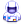Group: SE team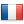France Messages: 1016 Status: Offline Hi ! So I decided to start a topic about math, where we con discuss about numbers, functions and stuff, where we can find help for homework... I put here on the off-topic section, because I wanted to extend the subject to epistemology, logic and philosophy. So I'll start with one question, I'm interrested in your thoughts about. So, do you think math was invented or discovered ? I've been thinking about this since a long time, and I want to find a solution without contradictions. Want some music of mine ? Please go here !RockoRocks Date: Monday, 02.12.2013, 20:25 | Message # 2World Builder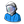Group: Users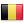Belgium Messages: 674 Status: Offline Discovered (I think) ... 1 + 1 = 2 no matter where you go... I will be inactive on this forum for the time being. Might come back eventually AMD AR-3305M APU w/ Radeon HD 1.90 GHz 6,00 GB RAM
SalvoDate: Monday, 02.12.2013, 20:46 | Message # 3Star Engineer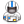Group: Local Moderators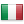Italy
Messages: 1400
Status: Offline
do you think math was invented or discovered ?

I think math was invented, as a way to represent numbers, calculations, functions etc.

What I like of math is that if we will meet aliens someday, they could have a similar way to represent numbers, so if we teach them our signs, we could easily share mathematics and physics formulas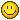The universe is not required to be in perfect harmony with human ambition.

CPU: Intel Core i7 4770 GPU: ASUS Radeon R9 270 RAM: 8 GBs

(still don't know why everyone is doing this...)

Edited by Salvo - Tuesday, 03.12.2013, 10:33Fireinthehole- Date: Monday, 02.12.2013, 20:57 | Message # 4Pioneer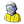Group: Translators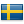Sweden Messages: 365 Status: Offline If it was invented then there should be more I think. Then the rules of math would be different in different cultures, and it would be possible to divide by 0 because it's just an invention and not a discovery. Love Space Engine!midtskogen Date: Monday, 02.12.2013, 21:34 | Message # 5Star EngineerGroup: Users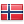Norway Messages: 1674 Status: Offline Math is invented. It's the tool we need to reason about discoveries. Quote Fireinthehole ()If it was invented then there should be more I think. Then the rules of math would be different in different cultures, and it would be possible to divide by 0 because it's just an invention and not a discovery. No. The different cultures have faced the same problems, so it's not very surprising that they arrive more or less at the same tools. And you don't make tools that don't have an application (like division by 0). NIL DIFFICILE VOLENTIEdited by midtskogen - Monday, 02.12.2013, 21:34
 DeathStar Date: Monday, 02.12.2013, 21:35 | Message # 6 PioneerGroup: Users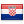Croatia Messages: 515 Status: Offline Really difficult question, because it can be viewed from many different angles. To me, a "discovery" is when the existence of a certain object, force or anything really that hasn't been known before, is acknowledged by a human being. An "invention" is when a previously non-existent object, force or anything is made by a human being. That is where the problem lies- math is somewhere between those two. Although it is present everywhere in nature, it is a system as well, that is made by humans. To me, math was invented, while mathematical laws were discovered.
 Watsisname Date: Monday, 02.12.2013, 22:13 | Message # 7Galaxy Architect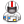Group: Global Moderators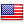United States Messages: 2613 Status: Offline To me, math was invented, while mathematical laws were discovered. I concur with this.The universe is the way it is and it operates under a set of laws, we might say the laws of physics or nature. We have descriptions or models of these phenomena that are mathematical in form, and they seem to work very well. But the mathematics itself is invented, while the laws are discovered. Edit: I should also distinguish between the above physical laws and mathematical 'laws', which are better called theorems (e.g. Pythagorean theorem, or fundamental theorem of calculus). They are tautologies, meaning they are fundamentally true because of the logic being applied. We may invent or define the rules, and the theorems are 'discovered' as a result of these rules.Voekoevaka Date: Tuesday, 03.12.2013, 00:46 | Message # 8World BuilderGroup: SE teamFrance Messages: 1016 Status: Offline Almost your opinions are like mine (and I'm surprised, I had the same discussion with friends, and they all thought math is a pure invention). There exist mathematical truths, which are absolute (example : the natural numbers : this concept is really fundamental), and these mathematical concepts were discovered by our civilization. But humans needs something to represent themselves these concepts, so they invented notations, which are part of the math. So, we have fundamental laws, and their human interpretation. With modern math, it is quite difficult to discern what is discovered and what is invented. For example, we have choose the decimal system to represent real numbers. But, in mathematics, it exists every possible system. So the "invented" part is the choice we made of using figures from 0 to 9 to represent values. Imagine math like an infinite book, where we pickup some notions and study them according to fundamental mathematical laws. verything exist in math, and everything could be discovered, after inventing notations, we choose what is interresting to study, and what is useless to study, so this new "invented part" is the choice of what should be studied in math. Quote Fireinthehole ()If it was invented then there should be more I think. Then the rules of math would be different in different cultures, and it would be possible to divide by 0 because it's just an invention and not a discovery. This is kind off-topic. Under certain conditions, we could define mathematically a division by 0, and there are no cultural aspects on that, except the notations. Division by 0 is an universal problem. Math is invented. It's the tool we need to reason about discoveries. Not only. Mathematicians were building theories with no points on stydying physics. Some of them were used much after (like complex numbers in electricity, differential manifolds in general relativity). And there's still a lot of mathematical laws which are unusued on other sciences.I think math was invented, as a way to represent numbers, calculations, functions etc. I guess you dealt with math notations. What I like of math is that if we will meet aliens someday, they could have a similar way to represent numbers, so if we teach them our signs, we could easily share mathematics and physics formulas So it is universal, and so it must be a discovery. Want some music of mine ? Please go here !midtskogen Date: Tuesday, 03.12.2013, 06:59 | Message # 9Star EngineerGroup: UsersNorway Messages: 1674 Status: Offline There exist mathematical truths, which are absolute (example : the natural numbers : this concept is really fundamental), and these mathematical concepts were discovered by our civilization. Names like Peano and Bertrand Russell immediately pop up in my mind when you say that. There exist lengthy discussions on this, perhaps the Principia Mathematica. No, natural numbers aren't regarded as god given or anything like that in modern mathematics. You can easily define natural numbers by the number 0 and the successor function S(x) of a number. Bottom line: we define axioms, we do not discover them. With them we form tautologies. EDIT: By defining (or inventing) the axioms of math one can imagine that as creating a mathematical universe in which there are laws to be discovered. But they are really just consequences of the tautology that you created, ultimately corollaries of the axioms, aren't they? Kind of like, if you're God and you create the universe, would you be an inventor of the physical laws within it or a discoverer of them? For instance, if we define natural numbers using Peano axioms (0 and S(x)) and define addition this way: x + 0 = x [A1] x + S(y) = S(x + y) [A2], we can prove the law of associativity, but is it really a discovery? So, we want to prove (x + y) + z = x + (y + z) and we can do it by induction on z: So, the case z = 0 first: (x + y) + 0 = x + y (by A1) = x + (y + 0) (also by A1) For the induction step on z our induction hypothesis will be: (x + y) + z = x + (y + z) We can then write: (x + y) + S(z) = S((x + y) + z) (by A2) = S(x + (y + z)) (by the induction hypothesis) = x + S(y + z) (by A2) = x + (y + S(z)) (by A2) QED. We only used the axioms that we invented. Did we really discover anything? NIL DIFFICILE VOLENTIEdited by midtskogen - Tuesday, 03.12.2013, 14:38
 Voekoevaka Date: Tuesday, 03.12.2013, 10:04 | Message # 10World BuilderGroup: SE teamFrance Messages: 1016 Status: Offline You can easily define natural numbers by the number 0 and the successor function S(x) of a number. Yes, this is axiom of infinity. But I think there is a more fundamental reason for the existence of natural numbers. It seems that in modern mathematics, we have created axioms to justify the existance, but there are plenty of ways to define such a concept. Axioms are just a kind of procedure we made to put the set theory as the base of mathematics. There are a lot of others ways to define the mathematics, but we can't imagine talking about math without dealing with natural numbers. To resume what I have said, axioms were invented, as a way to understand the fundamental objects like natural numbers (this is just my opinion). So axioms are not the most fundamental part of mathematics, they are just a point of view. Want some music of mine ? Please go here !midtskogen Date: Tuesday, 03.12.2013, 10:22 | Message # 11Star EngineerGroup: UsersNorway Messages: 1674 Status: Offline Yes, this is axiom of infinity. Not sure if I get that, but, yes, simple recursive definitions imply infinity, which is what we want for natural numbers. Interestingly, this brings us to the question whether natural numbers actually exist in the real world or whether natural numbers are simply an abstract construction. Does infinity exist? But I think there is a more fundamental reason for the existence of natural numbers. It seems that in modern mathematics, we have created axioms to justify the existance, but there are plenty of ways to define such a concept. Axioms are just a kind of procedure we made to put the set theory as the base of mathematics. Math doesn't like the concept of things existing simply because they evidently do, or anything that are so because they simply are so. So we base math on axioms, make axioms even for natural numbers. A supposed deeper meaning of numbers then doesn't belong to math. NIL DIFFICILE VOLENTIEdited by midtskogen - Tuesday, 03.12.2013, 10:27
 Voekoevaka Date: Tuesday, 03.12.2013, 10:38 | Message # 12World BuilderGroup: SE teamFrance Messages: 1016 Status: Offline Yes, I know, this belongs to epistemology. But natural numbers are in the structure of reality itself, everything is countable. Defining mathematically like you do on your edit with sucessor function is an invention, as it is an interpretaton. Want some music of mine ? Please go here !Aerospacefag Date: Tuesday, 03.12.2013, 15:29 | Message # 13PioneerGroup: Users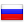Russian Federation Messages: 401 Status: Offline But natural numbers are in the structure of reality itself, everything is countable. Defining mathematically like you do on your edit with sucessor function is an invention, as it is an interpretaton. If we would try to count how many times math has changed to accommodate the complexity of reality, we probably would have stopped by the beginning of 20th century. Even the concept of non-euclidean geometry is so alien to the common sense, we wold probably never knew it before 18 century, when it was invented. There is even a sort of numbers that were deliberately called irrational, because they confront our "rational" thinking. As well as matrices, imaginary numbers, integral equations and function imagining. And tensors, of which I have no positive knowledge, unfortunately.
 Voekoevaka Date: Tuesday, 03.12.2013, 21:08 | Message # 14World BuilderGroup: SE teamFrance Messages: 1016 Status: Offline If we would try to count how many times math has changed to accommodate the complexity of reality, There are two points I disagree in this sentence. Firstly, the example you give of non-euclidian was not really a change in mathematics, but it was an extension of the knowledge. Old theorems aren't replaced by news, as they're still right. In phisics, theories are just models, so they could be dismissed. And secondly, you consider math just as a tool for physicians, everything in math was not made to accomodate the complexity in reality. But I agree with you with the fact that maths were often against common sense. When Cantor discovered there are different sizes of infinities, the rest of the mathematicians were all skeptic. But we know today his theory is true. Want some music of mine ? Please go here !Aerospacefag Date: Tuesday, 03.12.2013, 23:24 | Message # 15PioneerGroup: UsersRussian Federation Messages: 401 Status: Offline Firstly, the example you give of non-euclidian was not really a change in mathematics, but it was an extension of the knowledge. Indeed it was a change that removed euclidean mathematic from the point of center of the Universe to a position mere special case, albeit with preservation of all the axioms, like it was made for Newtonian physics later. And yes I recognize math as a tool, although it is the ultimate tool of mind, so that all other disciplines is a mere application of that tool to different sides of life, be that physics or account management.
 Forum » SpaceEngine » Off-topic Discussions » Math discussions (All math should go here)
• Page 1 of 1
• 1
 Search: